ShymeanV0.7.0

<!--more-->

1. 梳理游戏逻辑• 开始游戏后，鸟受重力影响会下降，点击屏幕可以让鸟向上飞行一段距离，鸟撞击到地面则游戏结束
• 整个场景由右向左移动，每隔一段时间会生成上下两根管道，需要控制鸟通过管道中间的空白区域，鸟撞击到管道则游戏结束
• 每通过一根管道，则积分+1，游戏目标是尽可能获得多的积分

• 鸟，玩家控制的主角
• 管道，上下成对出现，水平方向移动
• 地面，水平方向移动
• 分数

• 游戏流程，如开始、暂停、逻辑等
• 物理系统，主要是竖直方向上的重力系统
• 碰撞检测，鸟与管道、鸟与地面
• 一些动画，鸟排翅动画、鸟向上飞行动画、地面水平移动动画
• 预制体与对象池，需要随机生成管道，当管道从屏幕上消失之后可以回收复用
• 组件封装，将不同的逻辑封装在不同的组件中

2. 初始化项目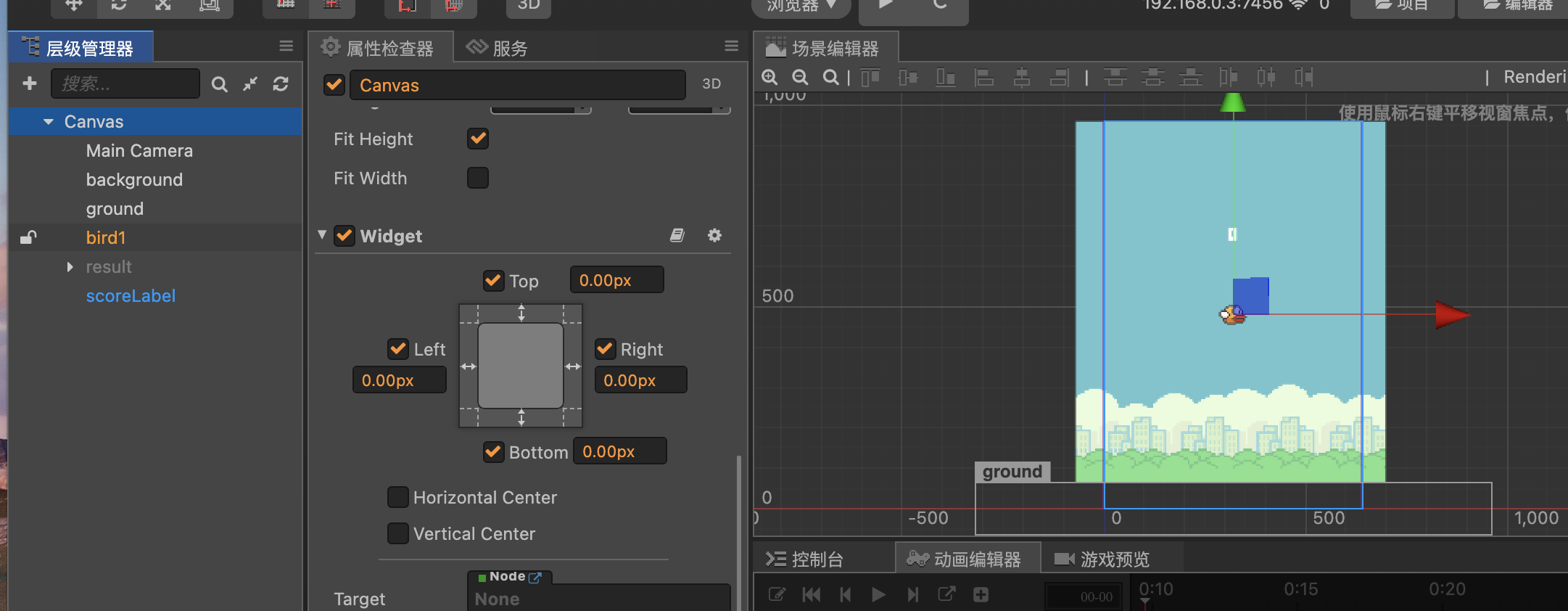game组件会维持对其他脚本组件的引用，这样就可以很方便地调用其他组件的方法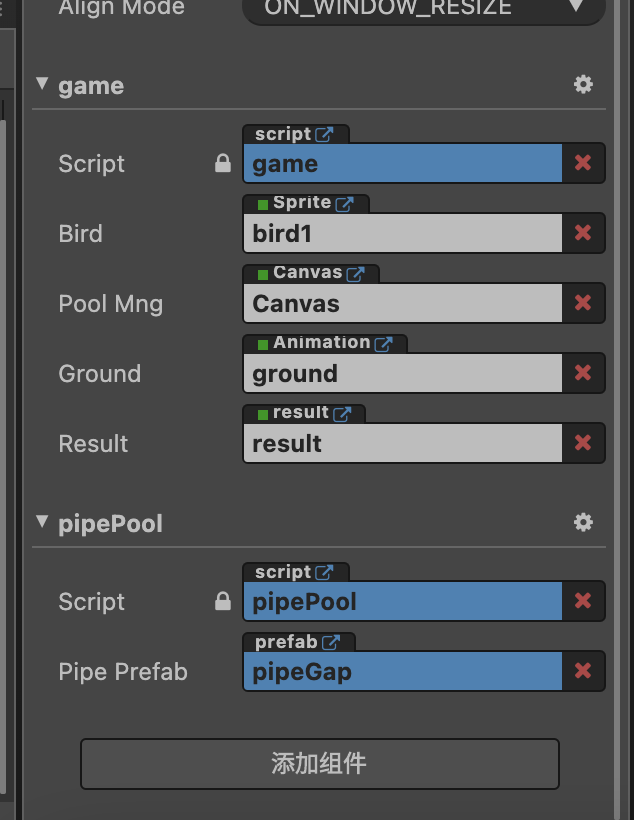3. 物理系统和碰撞系统

initPhysicsManager() {
const instance = cc.director.getPhysicsManager()
instance.enabled = true
// instance.debugDrawFlags = 4 // 调试模式
instance.gravity = this.gravity;
const collisionManager = cc.director.getCollisionManager();
collisionManager.enabled = true
}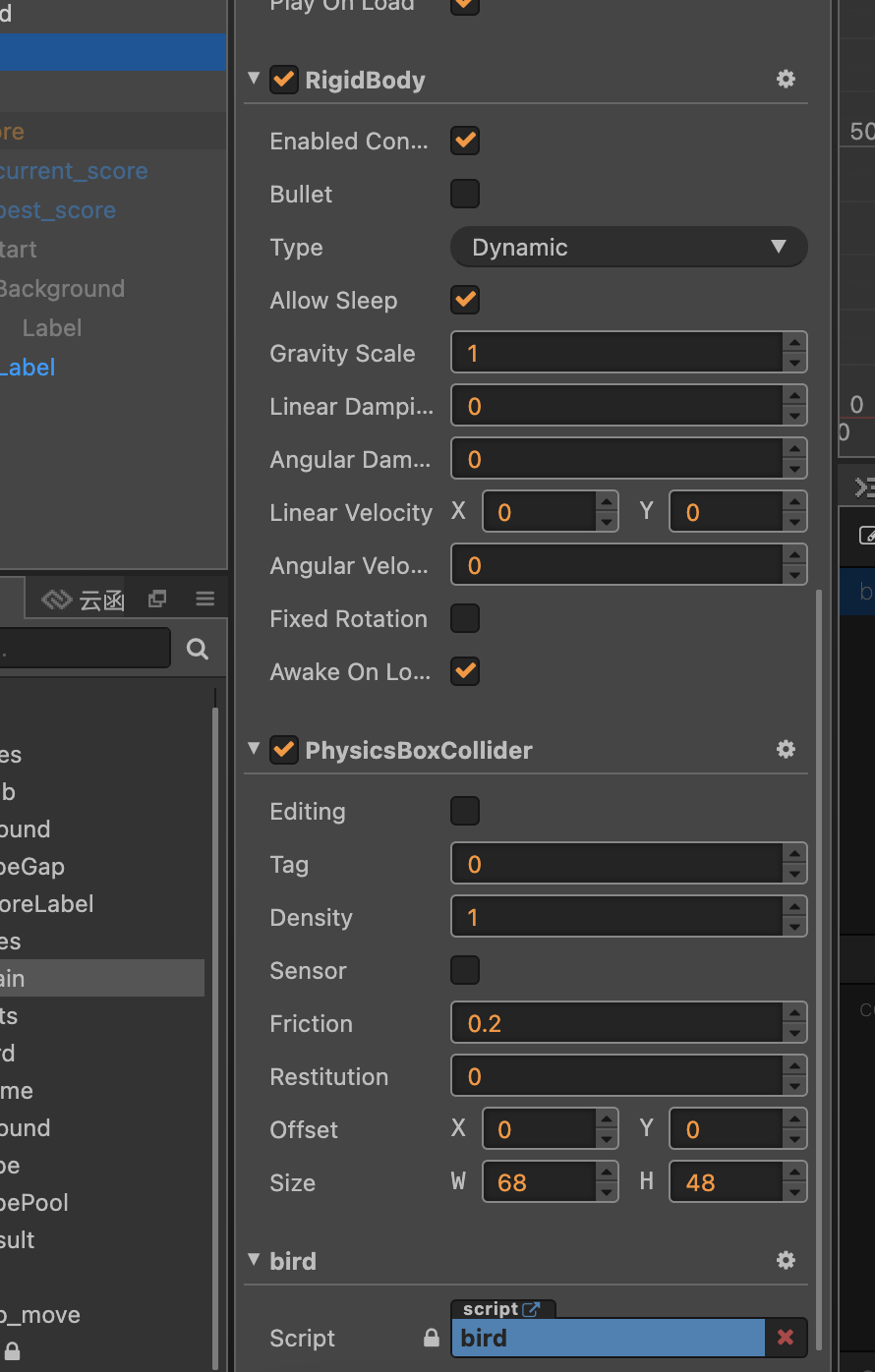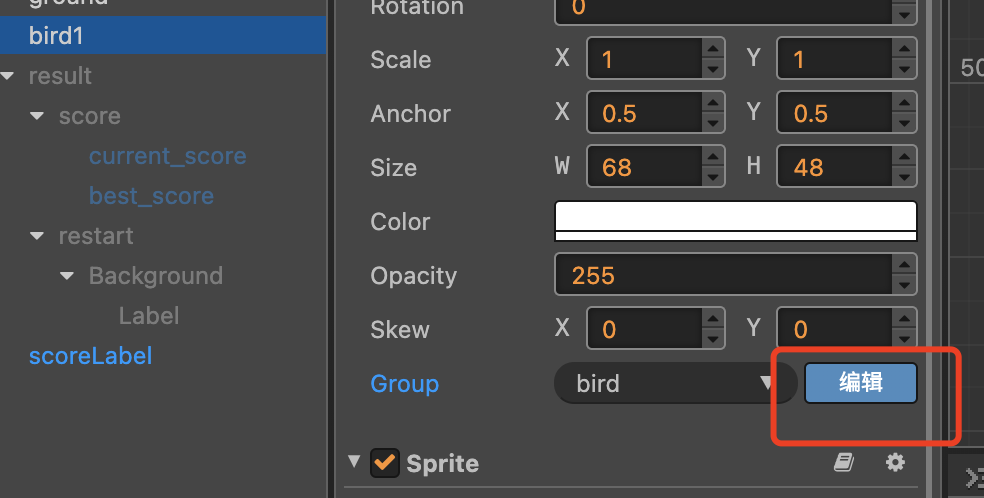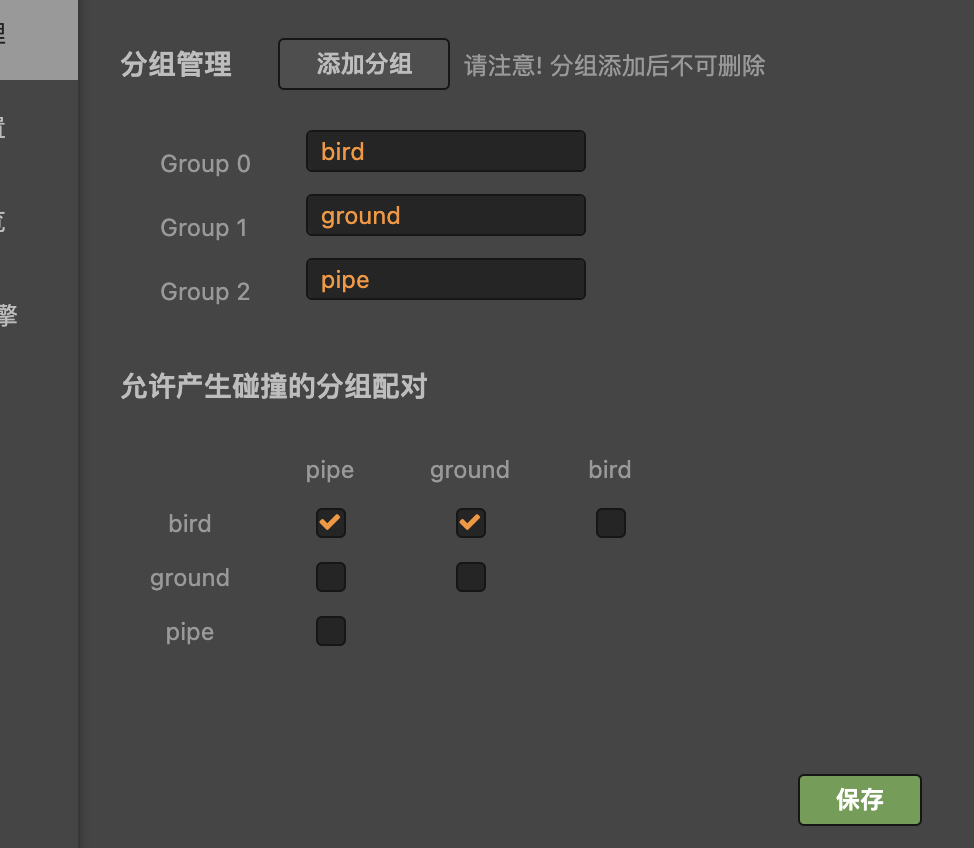4. 鸟

@ccclass
export default class NewClass extends cc.Component {
// 只在两个碰撞体开始接触时被调用一次
onBeginContact(contact, selfCollider, otherCollider): void {
this.game.gameOver()
}
}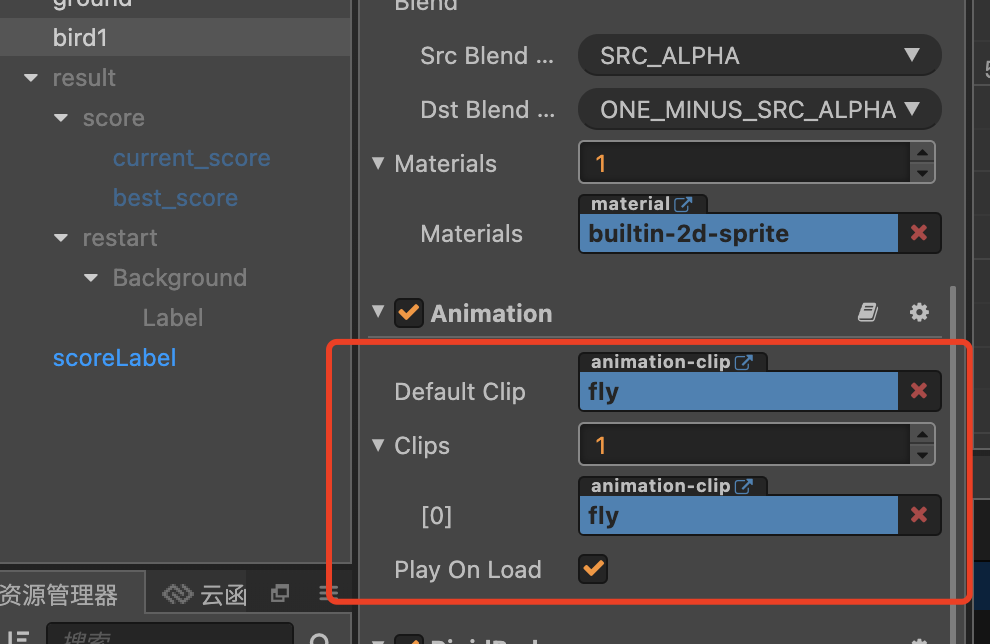fly() {
const body = this.node.getComponent(cc.RigidBody)
// 飞行时关闭重力
body.type = cc.RigidBodyType.Static
this.node.angle = 30

this.node.stopAction(this.lastAction)
this.lastAction = cc.sequence(
cc.sequence(
cc.moveBy(this.jumpDuration, cc.v2(0, this.jumpHeight)).easing(cc.easeSineOut()),
cc.callFunc(function (target) {
// 重新开启重力
body.type = cc.RigidBodyType.Dynamic
}, this)
),
cc.rotateTo(this.jumpDuration, 90).easing(cc.easeIn(1.2)), // 向下俯冲
);

this.node.runAction(this.lastAction)
}

this.node.on(cc.Node.EventType.MOUSE_DOWN, () => {
this.bird.fly()
})

5. 管道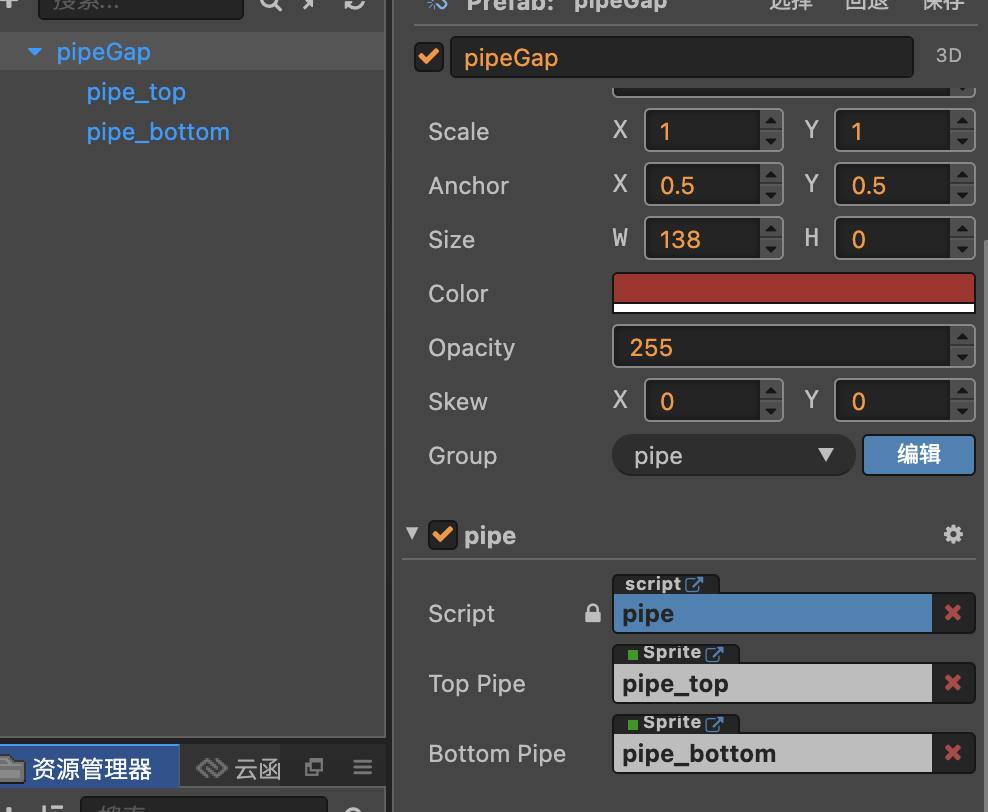5.1. 移动

update(dt) {
const speed = this.game.speed * dt
this.node.x -= speed

// 刚体需要手动同步
this.topPipe.getComponent(cc.RigidBody).syncPosition(true);
this.bottomPipe.getComponent(cc.RigidBody).syncPosition(true);

if (!this.isPass && this.game && this.game.bird.node.x > this.node.x) {
this.isPass = true
this.game.passPipe()
this.game.createPipe()
}
}

5.2. 随机尺寸算法

const random = (min, max) => {
return Math.random() * (max - min) + min
}
initPos() {
this.node.setPosition(cc.v2(360, 0));

const groundH = 64
const screenH = 960

let gap = random(200, 300) // 可以通过的区域
let h1 = random(200, 400) // 顶部管道高度，通过顶部管道和可通过区域，就可以计算出底部管道的高度
// let gap = 150
// let h1 = 300

let y1 = (screenH / 2 - h1) + h1 / 2

this.topPipe.node.height = h1
this.topPipe.node.y = y1

// h1 + h2 + gap = screenH - groundH
let h2 = screenH - groundH - h1 - gap

// y2 + h2/2 = screenH/2 - groundH
let y2 = screenH / 2 - groundH - h2 / 2

this.bottomPipe.node.height = h2
this.bottomPipe.node.y = -y2
}

5.3. 管道生成器

@ccclass
export default class NewClass extends cc.Component {

@property(cc.Prefab)
pipePrefab: cc.Prefab

pool: cc.NodePool = null
nodeList: cc.Node[]
game: Game

init(game: Game) {
this.game = game
this.nodeList = []
this.initPool()
}

initPool() {
this.pool = new cc.NodePool();
let initCount = 3; // 最大节点，3个
for (let i = 0; i < initCount; ++i) {
let node = cc.instantiate(this.pipePrefab); // 创建节点
this.pool.put(node); // 通过 put 接口放入对象池
this.nodeList.push(node)
}
}

// 从节点池获取一个节点
createPipe() {
let pipe = null
if (this.pool.size() > 0) {
pipe = this.pool.get()
} else {
pipe = cc.instantiate(this.pipePrefab)
}
pipe.getComponent("pipe").init(this.game)

return pipe
}

// 回收节点，在合适的时机调用
reusePipe(pipe: cc.Node) {
this.pool.put(pipe)
}

reset() {
this.nodeList.forEach(node => {
this.reusePipe(node)
})
}
}

// pipe.ts
update(dt) {
// ... 其他逻辑

// 回收
if (this.isPass && this.node.x <= -400) {
this.game.poolMng.reusePipe(this.node)
}

}

6. 地面

// 初始化滴管
initGround() {
for (let i = -18; i <= 18; ++i) {
let tile = cc.instantiate(this.groundTile);
tile.x = i * 37
}
}

update(dt) {
// 地面移动动画
const speed = this.game.speed * dt
this.node.x -= speed
if (this.node.x < -320) {
this.node.x += 320 - 18.5
}
}

7. 游戏结果

// result.ts
}

this.score = num
this.scoreLabel.string = this.score.toString()
}

showResult() {
this.maxScore = Math.max(this.maxScore, this.score)

this.scoreLabel.node.active = false

this.node.active = true
this.node.zIndex = 9999

this.currentScoreLabel.string = this.score.toString()
this.bestScoreLabel.string = this.maxScore.toString()

this.scoreLabel.node.active = false
}

// game.ts
passPipe() {
}

gameOver() {
this.isOver = true

console.log('碰到障碍，游戏结束')
this.result.showResult()

cc.director.pause()
}

8. 小结

cocos虽然不是最适合做游戏的引擎，但是用来入门游戏开发还是很不错的。从比较简单的游戏入手，一步一步学习，总有一天可以做出心仪的游戏（对我自己说